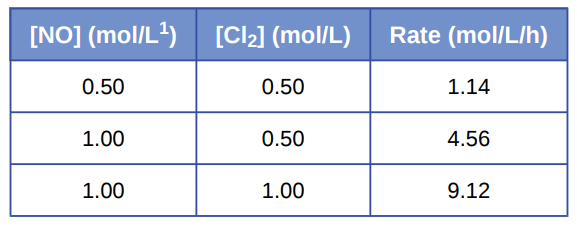# Problem: Nitrogen monoxide reacts with chlorine according to the equation:2NO(g) + Cl2 (g) ⟶ 2NOCl(g)The following initial rates of reaction have been observed for certain reactant concentrations:What is the rate equation that describes the rate’s dependence on the concentrations of NO and Cl2? What is the rate constant? What are the orders with respect to each reactant?

###### FREE Expert Solution

We’re being asked to calculate the rate law and the value of the rate constant (k) based on the reaction and data given.

Recall that the rate law only focuses on the reactant concentrations and has a general form of:

k = rate constant
A & B = reactants
x & y = reactant orders

We’re going to calculate the rate law and the rate constant using the following steps:

84% (14 ratings)###### Problem Details

Nitrogen monoxide reacts with chlorine according to the equation:

2NO(g) + Cl2 (g) ⟶ 2NOCl(g)

The following initial rates of reaction have been observed for certain reactant concentrations:What is the rate equation that describes the rate’s dependence on the concentrations of NO and Cl2? What is the rate constant? What are the orders with respect to each reactant?

Frequently Asked Questions

What scientific concept do you need to know in order to solve this problem?

Our tutors have indicated that to solve this problem you will need to apply the Rate Law concept. You can view video lessons to learn Rate Law. Or if you need more Rate Law practice, you can also practice Rate Law practice problems.

What professor is this problem relevant for?

Based on our data, we think this problem is relevant for Professor Delgado's class at FIU.

What textbook is this problem found in?

Our data indicates that this problem or a close variation was asked in Chemistry - OpenStax 2015th Edition. You can also practice Chemistry - OpenStax 2015th Edition practice problems.